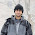Thursday, 25 July 2013

Poisson disc in Matlab

Poisson disc sampling is a nice way of getting random yet even coverage.

Following the helpful page from devmag here are 2d and 3d implementations of Poisson disc (sphere?) sampling in Matlab. Also an implementation using SPM's grey mask to parcellate grey matter to an arbitrary degree.

1 comment:

1.Hello,
In your 3D implementation of the Poisson disk sampling, when generating a new random point around a given point, you will have to use the following line
[x y z]=sph2cart(2*pi*rand(1),pi/2 - (pi*rand(1)),min_dist*(rand(1)+1));